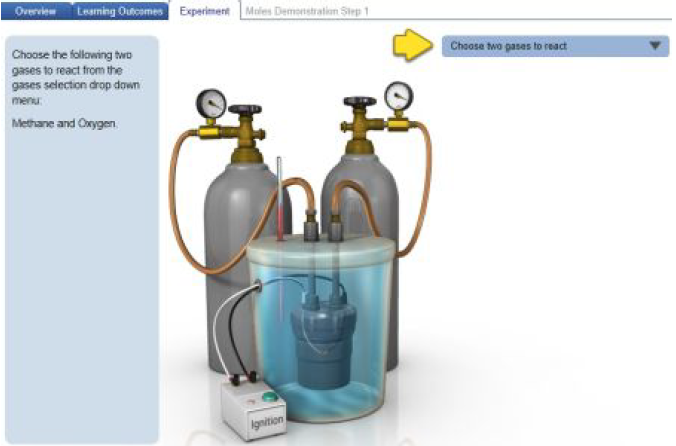# Problem: Based on the ideal gas law, there is a simple equivalency that exists between the amount of gas and the volume it occupies. At standard temperature and pressure (STP; 273.15 K and 1 atm, respectively), one mole of gas occupies 22.4 L of volume.What mass of methanol (CH3OH) could you form if you reacted 5.39 L of a gas mixture (at STP) that contains an equal number of carbon monoxide (CO) and hydrogen gas (H2) molecules?Express the mass in grams to three significant digits.Carbon monoxide (CO) can react with hydrogen gas (H2) to form methanol (CH3OH) as shown in the Carbon Monoxide and Hydrogen - Sample 2 reaction in the simulation. The same reactants can also form methane (CH4) and water (H2O) as shown in the Carbon Monoxide and Hydrogen - Sample 1 reaction in the simulation. The latter reaction (Sample 1) is actually more commonly employed in reverse as a method for producing hydrogen gas, a process known as steam reforming, but it requires a lot of heat.For the following exercises, you can track and confirm the amounts of reactants and products (in both moles and mass) using the Run Experiment tool under the Experiment tab in the simulation. Note that the simulation checks your values to a different number of significant digits than this tutorial. We will explore these two reactions of carbon monoxide and hydrogen.

###### FREE Expert SolutionView Complete Written Solution
###### Problem Details

Based on the ideal gas law, there is a simple equivalency that exists between the amount of gas and the volume it occupies. At standard temperature and pressure (STP; 273.15 K and 1 atm, respectively), one mole of gas occupies 22.4 L of volume.

What mass of methanol (CH3OH) could you form if you reacted 5.39 L of a gas mixture (at STP) that contains an equal number of carbon monoxide (CO) and hydrogen gas (H2) molecules?

Express the mass in grams to three significant digits.

Carbon monoxide (CO) can react with hydrogen gas (H2) to form methanol (CH3OH) as shown in the Carbon Monoxide and Hydrogen - Sample 2 reaction in the simulation. The same reactants can also form methane (CH4) and water (H2O) as shown in the Carbon Monoxide and Hydrogen - Sample 1 reaction in the simulation. The latter reaction (Sample 1) is actually more commonly employed in reverse as a method for producing hydrogen gas, a process known as steam reforming, but it requires a lot of heat.

For the following exercises, you can track and confirm the amounts of reactants and products (in both moles and mass) using the Run Experiment tool under the Experiment tab in the simulation. Note that the simulation checks your values to a different number of significant digits than this tutorial. We will explore these two reactions of carbon monoxide and hydrogen.